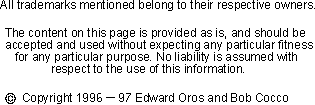The standard ground plane antenna consists of a vertical radiator which is insulated and centered between 4 horizontal surrounding radials which extend out from the base of the antenna. Each radial is evenly spaced (90 degrees apart from each other) around the vertical element.

All antenna lengths depend on various factors. Some of these factors are:the height above ground, the diameter of the wire, nearby structures, the effects of other antennas in the area and even the conductivity of the soil. (Because of the artificial ground provided by the radials, the height above ground should have little effect on this type of antenna.)

This page allows you to calculate the length for a quarter wave ground plane antenna. It uses the standard formula, 234/f MHz to calculate the element lengths. If you've experimented with ground planes before and know of a better formula for your QTH, feel free to change the formula to suit.
Enter the formula for the antenna calculation

Divided by Freq MHz

Your ground plane's vertical length is feet
Each of the 4 radials of the ground plane is also feet

Back to the Antenna Elmer